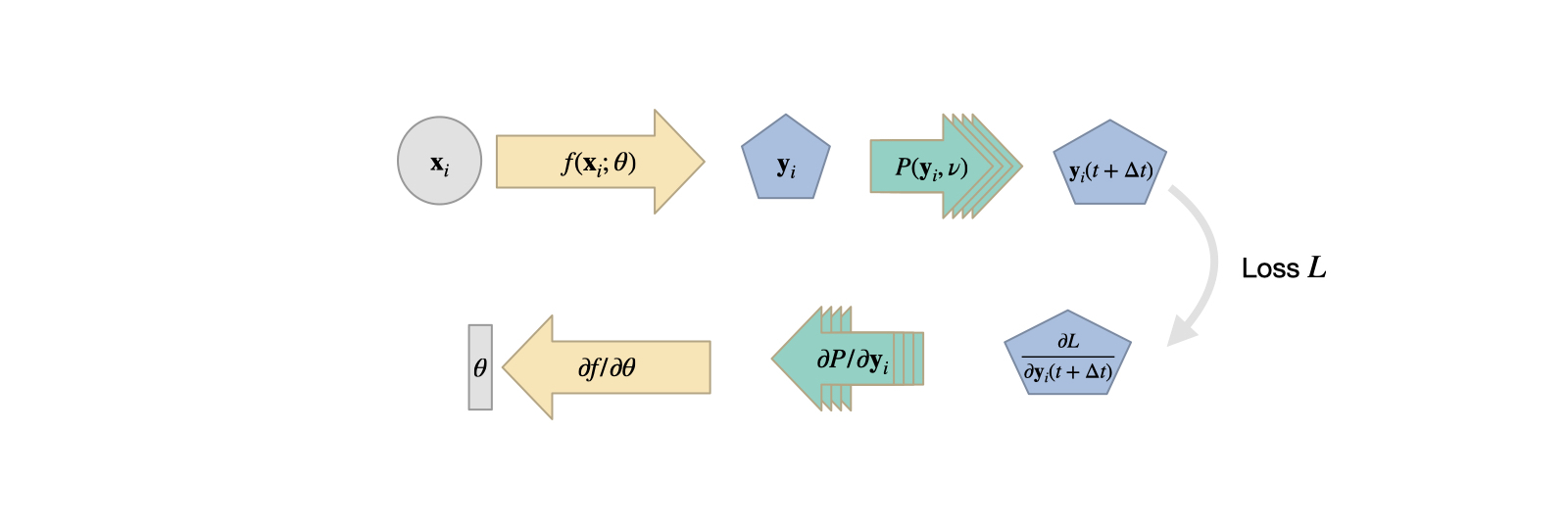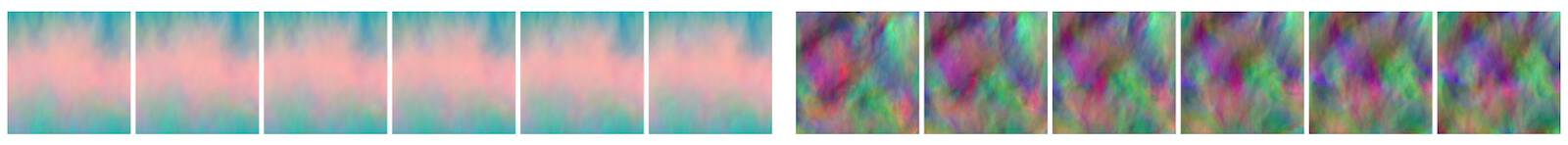# Discussion¶

To summarize, the training via differentiable physics (DP) as described so far allows us to integrate full numerical simulations into the training of deep neural networks. As a consequence, this let’s the networks learn to interact with these simulations. While we’ve only hinted at what could be achieved via DP approaches it is nonetheless a good time to discuss some additional properties, and summarize the pros and cons.## Time steps and iterations¶

When using DP approaches for learning applications, there is a lot of flexibility w.r.t. the combination of DP and NN building blocks. As some of the differences are subtle, the following section will go into more detail. We’ll especially focus on solvers that repeat the PDE and NN evaluations multiple times, e.g., to compute multiple states of the physical system over time.

To re-cap, here’s the previous figure about combining NNs and DP operators. In the figure these operators look like a loss term: they typically don’t have weights, and only provide a gradient that influences the optimization of the NN weights:Fig. 11 The DP approach as described in the previous chapters. A network produces an input to a PDE solver $$\mathcal P$$, which provides a gradient for training during the backpropagation step.

This setup can be seen as the network receiving information about how it’s output influences the outcome of the PDE solver. I.e., the gradient will provide information how to produce an NN output that minimizes the loss. Similar to the previously described physical losses (from Physical Loss Terms), this can mean upholding a conservation law.

Switching the Order

However, with DP, there’s no real reason to be limited to this setup. E.g., we could imagine a swap of the NN and DP components, giving the following structure:Fig. 12 A PDE solver produces an output which is processed by an NN.

In this case the PDE solver essentially represents an on-the-fly data generator. This is not necessarily always useful: this setup could be replaced by a pre-computation of the same inputs, as the PDE solver is not influenced by the NN. Hence, we could replace the $$\mathcal P$$ invocations by a “loading” function. On the other hand, evaluating the PDE solver at training time with a randomized sampling of the parameter domain of interest can lead to an excellent sampling of the data distribution of the input, and hence yield accurate and stable NNs. If done correctly, the solver can alleviate the need to store and load large amounts of data, and instead produce them more quickly at training time, e.g., directly on a GPU.

Time Stepping

In general, there’s no combination of NN layers and DP operators that is forbidden (as long as their dimensions are compatible). One that makes particular sense is to “unroll” the iterations of a time stepping process of a simulator, and let the state of a system be influenced by an NN.

In this case we compute a (potentially very long) sequence of PDE solver steps in the forward pass. In-between these solver steps, an NN modifies the state of our system, which is then used to compute the next PDE solver step. During the backpropagation pass, we move backwards through all of these steps to evaluate contributions to the loss function (it can be evaluated in one or more places anywhere in the execution chain), and to backpropagte the gradient information through the DP and NN operators. This unrolling of solver iterations essentially gives feedback to the NN about how it’s “actions” influence the state of the physical system and resulting loss. Due to the iterative nature of this process, many errors increase exponentially over the course of iterations, and are extremely difficult to detect in a single evaluation. In these cases it is crucial to provide feedback to the NN at training time who the errors evolve over course of the iterations. Note that in this case, a pre-computation of the states is not possible, as the iterations depend on the state of the NN, which is unknown before training. Hence, a DP-based training is crucial to evaluate the correct gradient information at training time.Fig. 13 Time stepping with interleaved DP and NN operations for $$k$$ solver iterations. The dashed gray arrows indicate optional intermediate evaluations of loss terms (similar to the solid gray arrow for the last step $$k$$).

Note that in this picture (and the ones before) we have assumed an additive influence of the NN. Of course, any differentiable operator could be used here to integrate the NN result into the state of the PDE. E.g., multiplicative modifications can be more suitable in certain settings, or in others the NN could modify the parameters of the PDE in addition to or instead of the state space. Likewise, the loss function is problem dependent and can be computed in different ways.

DP setups with many time steps can be difficult to train: the gradients need to backpropagate through the full chain of PDE solver evaluations and NN evaluations. Typically, each of them represents a non-linear and complex function. Hence for larger numbers of steps, the vanishing and exploding gradient problem can make training difficult (see Reducing Numerical Errors with Deep Learning for some practical tips how to alleviate this).

## Alternatives: noise¶

It is worth mentioning here that other works have proposed perturbing the inputs and the iterations at training time with noise [SGGP+20] (somewhat similar to regularizers like dropout). This can help to prevent overfitting to the training states, and hence shares similarities with the goals of training with DP.

However, the noise is typically undirected, and hence not as accurate as training with the actual evolutions of simulations. Hence, this noise can be a good starting point for training that tends to overfit, but if possible, it is preferable to incorporate the actual solver in the training loop via a DP approach.## Summary¶

To summarize the pros and cons of training NNs via DP:

✅ Pro:

• Uses physical model and numerical methods for discretization.

• Efficiency and accuracy of selected methods carries over to training.

• Very tight coupling of physical models and NNs possible.

❌ Con:

• Not compatible with all simulators (need to provide gradients).

• Require more heavy machinery (in terms of framework support) than previously discussed methods.

Here, the last negative point (regarding heavy machinery) is bound to strongly improve in a fairly short amount of time. However, for now it’s important to keep in mind that not every simulator is suitable for DP training out of the box. Hence, in this book we’ll focus on examples using phiflow, which was designed for interfacing with deep learning frameworks.

Next we can target more some complex scenarios to showcase what can be achieved with differentiable physics. This will also illustrate how the right selection of numerical methods for a DP operator yields improvements in terms of training accuracy.